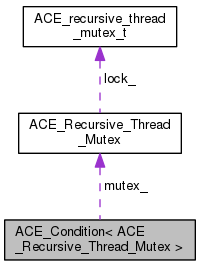ACE  6.3.0
ACE_Condition< ACE_Recursive_Thread_Mutex > Class Template Reference

ACE_Condition template specialization written using ACE_Recursive_Thread_Mutex. This allows threads to block until shared data changes state using recursive mutexes. More...

`#include <Condition_Recursive_Thread_Mutex.h>`

Collaboration diagram for ACE_Condition< ACE_Recursive_Thread_Mutex >:[legend]

## Public Member Functions

Initialize the condition variable with a recursive mutex. More...

ACE_Condition (ACE_Recursive_Thread_Mutex &m, const ACE_Condition_Attributes &attributes)
Initialize the condition variable. More...

~ACE_Condition (void)
Implicitly destroy the condition variable. More...

int remove (void)

int wait (const ACE_Time_Value *abstime=0)

int wait (ACE_Recursive_Thread_Mutex &mutex, const ACE_Time_Value *abstime=0)

int signal (void)

Returns a reference to the underlying mutex;. More...

void dump (void) const
Dump the state of an object. More...

## Private Member Functions

void operator= (const ACE_Condition< ACE_Recursive_Thread_Mutex > &)

ACE_Condition (const ACE_Condition< ACE_Recursive_Thread_Mutex > &)

## Private Attributes

ACE_cond_t cond_
A normal (i.e., non-recursive) condition variable. More...

Reference to the recursive mutex. More...

## Detailed Description

### template<> class ACE_Condition< ACE_Recursive_Thread_Mutex >

ACE_Condition template specialization written using ACE_Recursive_Thread_Mutex. This allows threads to block until shared data changes state using recursive mutexes.

## Constructor & Destructor Documentation

Initialize the condition variable with a recursive mutex.

 ACE_Condition< ACE_Recursive_Thread_Mutex >::ACE_Condition ( ACE_Recursive_Thread_Mutex & m, const ACE_Condition_Attributes & attributes )

Initialize the condition variable.

 ACE_Condition< ACE_Recursive_Thread_Mutex >::~ACE_Condition ( void )

Implicitly destroy the condition variable.

private

## Member Function Documentation

 void ACE_Condition< ACE_Recursive_Thread_Mutex >::dump ( void ) const

Dump the state of an object.

Returns a reference to the underlying mutex;.

private
 int ACE_Condition< ACE_Recursive_Thread_Mutex >::remove ( void )

Explicitly destroy the condition variable. Note that only one thread should call this method since it doesn't protect against race conditions.

 int ACE_Condition< ACE_Recursive_Thread_Mutex >::signal ( void )

 int ACE_Condition< ACE_Recursive_Thread_Mutex >::wait ( const ACE_Time_Value * abstime = `0` )

Block on condition, or until absolute time-of-day has passed. If abstime == 0 use "blocking" <wait> semantics. Else, if abstime != 0 and the call times out before the condition is signaled <wait> returns -1 and sets errno to ETIME.

 int ACE_Condition< ACE_Recursive_Thread_Mutex >::wait ( ACE_Recursive_Thread_Mutex & mutex, const ACE_Time_Value * abstime = `0` )

Block on condition or until absolute time-of-day has passed. If abstime == 0 use "blocking" wait() semantics on the recursive mutex passed as a parameter (this is useful if you need to store the <Condition> in shared memory). Else, if abstime != 0 and the call times out before the condition is signaled <wait> returns -1 and sets errno to ETIME.